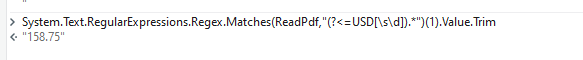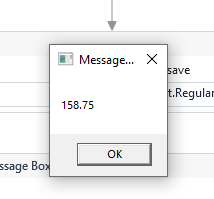# Need extract data rfom text file using Regex

I need to extract the below data from the attached text file.
Data : 158.75

pdf_text_file.txt (5.4 KB)

Hi,

Can you try the following expression?

``````System.Text.RegularExpressions.Regex.Match(strData,"(?<=TOTAL\s+AMOUNT\s+\(INCL\.\s+TAX\)\s+)[\d.,]+").Value
``````

Regards,

@shruthi_arali

Str_outRegex=System.Text.RegularExpressions.Regex.Match(Input,“Expression”).Value

1 Like

You can give this Regex Expression in Find Matching Patterns:

``````(?<=TOTAL\sAMOUNT(.*)\s)[\d.]+
``````

Use this syntax in Assign activity:

``````Matches= System.Text.RegularExpressions.Regex.Matches(yourstringinput,"(?<=TOTAL\sAMOUNT(.*)\s)[\d.]+")
``````

Datatype of Matches is IEnumerable(System.Text.RegularExpressions.Match)
Print it in a message box by using below syntax:
`Matches(0)`. This expression will extract an single value. If you have multiple values run a for each for matches.

Use this syntax in Assign activity. It will extract the first Matched value:

``````Matches= System.Text.RegularExpressions.Regex.Match(yourstringinput,"(?<=TOTAL\sAMOUNT(.*)\s)[\d.]+").Value
``````

Datatype of Matches is String
Print it in a message box by using below syntax:
`Matches.toString`

Hope it helps!!

1 LikeTry With this Expression

1 Like

Try this

`(?<=TOTAL\sAMOUNT\s\(INCL.\sTAX\)\s).*`

1 Like(Text.RegularExpressions.Regex.Matches(str,“(?<=Total Amount.*\s)\d+.+\d+”))(0).ToString
Try it

1 Like

(?<=[A-Z]+\s*([A-Z]+.\s*[A-Z]+)\s*)\d+.\d+Hey!Can u try it with this Regex Pattern

1 Like

This topic was automatically closed 3 days after the last reply. New replies are no longer allowed.